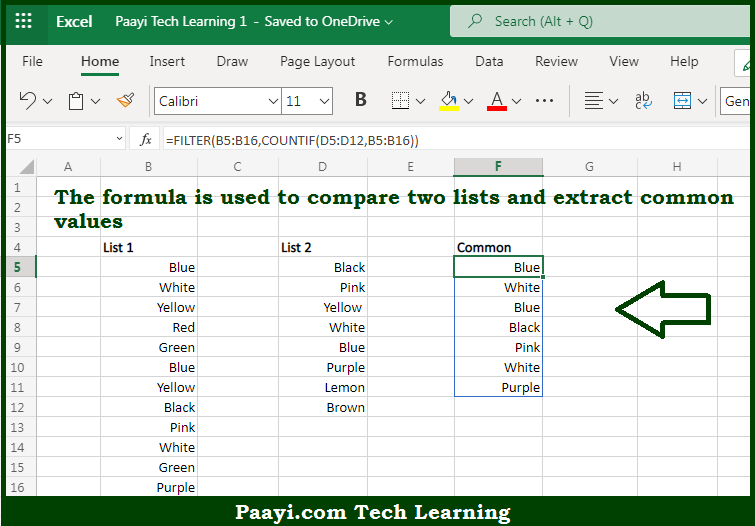# Learn How to Extract Common Values From Two Lists in Microsoft Excel

Written by | 0 Comments | 774 Views

In this article, you will learn how to evaluate things in Dynamic Arrays with formulas in Microsoft Excel using a single/combination(s) of functions. You will also know How to Extract Common Values From Two Lists and see the generic formula.

How to Extract Common Values From Two Lists in Microsoft Excel

The main purpose of this formula is to compare two lists and extract common values. Here we will learn how to extract common values from two lists in the workbook in Microsoft Excel. That implies, with the help of a formula based on the UNIQUE function you can able to compare two lists and extract common values. So, with the help of this formula, you can able to extract common values from two lists in the workbook in Microsoft Excel.

General Formula to Extract Common Values From Two Lists

=FILTER(list1,COUNTIF(list2,list1))

The Explanation for the Extract Common Values From Two ListsSo we know that with the help of the given formula above you can able to compare two lists and extract common values. Here we will learn how to extract common values from two lists in the workbook in Microsoft Excel. As we know that the  FILTER function only accepts an array of values and an "include" argument which filters the array based on a logical expression or value. So, with the help of this formula, you can able to compare two lists and extract common values. Here we will learn how to extract common values from two lists in the workbook in Microsoft Excel.